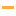Math Games

Play the best free online Math Games! Check out the Top 100 Math games from PrimaryGames.

#21Number Balls
#22Bus Driver's MathYou're the bus driver in this fun math game. Your job is to calculate whether the passengers have paid enough money for the bus fare.

#23RoboClockHelp RoboClock get through all five levels of this telling time game by selecting the right time.

#24Math Tiles: Thanksgiving Addition and SubtractionPractice addition and subtraction skills in this fun math game. Select from adding, subtracting or both and choose between a range of 10, 20, 100 or 1,000.

#25Math Car
#26Turkey Tens
#27Math Mosaics: Valentine's DayIt's color by number! To determine the color of each space solve each addition problem, then use the code at the side of the page. The final picture will be revealed as each square is filled.

#28Math Eggs: Prime NumbersCatch eggs from chickens that are prime numbers. Your score will be decreased if you catch composite numbers (not prime). You have 3 lives at the start of the game. Lose a life when an egg from a chicken showing a prime number breaks on the ground.

#29Two Minute Warning: Addition Flashcards - EasyPractice addition skills in this online flashcard game. You have 2 minutes to solve as many addition problems as you can. Use the keyboard or touchscreen to play. Are you looking to practice other math skills? Check out the entire Two Minute Warning line...

#30Skater Math
#31Math Riddles: ThanksgivingLook at each picture math equation. Use the information from equation to solve for the question mark. What number should replace the question mark?

#32Math Tiles: Autumn MultiplicationPractice multiplication skills with factors up to 12 in this fun puzzle game.

#33Two Minute Warning: Multiplication Flashcards - By 3Learn multiplication facts and how to multiply by 3 in this online flashcard game. You have 2 minutes to solve as many multiplication problems as you can. Use the keyboard or touchscreen to play and become a times table expert.

#34Times Tables DiplomaPractice multiplication and earn your Times Table Diploma! Learn all the multiplication facts and become a multiplication expert.

#35Counting Mosaics: AutumnIt's color by number! To determine the color of each space count up the number of dots, then use the code at the side of the page. The final picture will be revealed as each square is filled.

#36Math Mosaics: AutumnIt's color by number! To determine the color of each space solve each addition problem, then use the code at the side of the page. The final picture will be revealed as each square is filled.

#37The Number Game
#38Math Eggs: Factoring NumbersCatch eggs from chickens that are factors of the numbered label on the basket. Your score will be decreased if you catch numbers that are not factors. You have 3 lives at the start of the game. Lose a life when an egg from a chicken showing a correct fact

#39Two Minute Warning: Division Flashcards - EasyLearn division facts and how to divide by numbers from 1 to 5 in this online flashcard game. You have 2 minutes to solve as many division problems as you can. Use the keyboard or touchscreen to play and become a times table expert. Are you looking to...

#40Two Minute Warning: Multiplication Flashcards - By 2Learn multiplication facts and how to multiply by 2 in this online flashcard game. You have 2 minutes to solve as many multiplication problems as you can. Use the keyboard or touchscreen to play and become a times table expert.